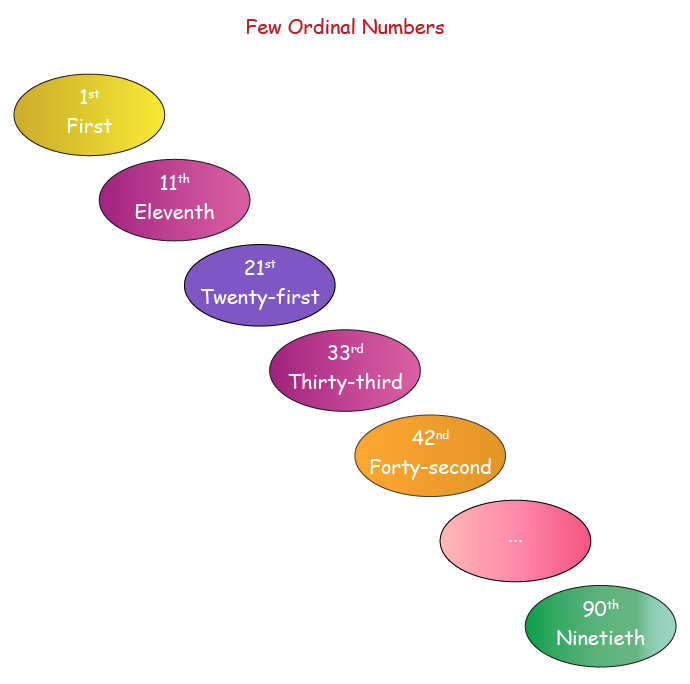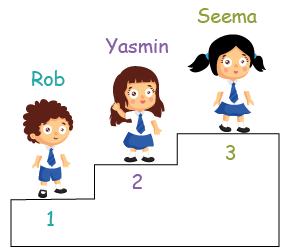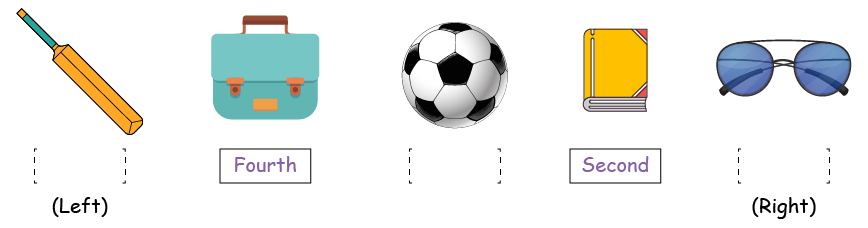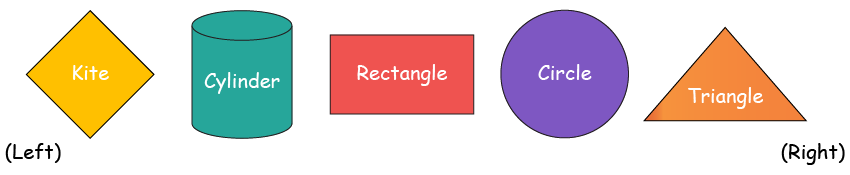# Ordinal Numbers

Ordinal Numbers
Go back to  'Numbers'

## Introduction

The cookies are kept in the $$3^\text{rd}$$ drawer from the top.
The orange dress is the $$7^\text{th}$$ one from the right.
The football is kept in the $$3^\text{rd}$$ carton box from the left.

All these sentences have one thing in common.
They all talk about the position of certain objects (cookies, orange dress, football).

This is the main element in the discussion of ordinal numbers.

## Ordinal Numbers

An ordinal number tells the numerical position of an object in a list. In other words, it indicates the order of things or objects, such as first, second, third, fourth, and so on.

An ordinal number usually ends in “th.”## Solved Examples

### Example 1

Look carefully at the picture, then answer the questions.(i)  Who is on the $$1^\text{st}$$ step?

(ii)  Yasmin is on which step?

(iii)  Seema is on Step 3. She is standing on which step?
Answer: $$3^\text{rd}$$ or third step

### Example 2

Look carefully at the picture, then answer the questions.(i)  Which object is $$5^\text{th}$$ from the right?

(ii)  In what position is the book from the left?
Answer: $$4^\text{th}$$ or fourth position

(iii)  In what position is the ball from the right?
Answer: $$3^\text{rd}$$ or third position

## Practice Questions

### Question 1

Look carefully at the picture, then answer the questions.(i)  In which position from the left is the shape that is $$4^\text{th}$$ from the right?

(ii)  How many shapes are to the right of the shape that is $$5^\text{th}$$ from the left?

(iii)  Which shape is in the $$4^\text{th}$$ position from the left?

(iv)  Which shape is in the $$3^\text{rd}$$ position from the right?

(v)  Is it possible to have exactly one shape in the middle of this entire set, without adding or removing a shape? Explain.

More Important Topics
Numbers
Algebra
Geometry
Measurement
Money
Data
Trigonometry
Calculus
More Important Topics
Numbers
Algebra
Geometry
Measurement
Money
Data
Trigonometry
Calculus
Learn from the best math teachers and top your exams

• Live one on one classroom and doubt clearing
• Practice worksheets in and after class for conceptual clarity
• Personalized curriculum to keep up with school